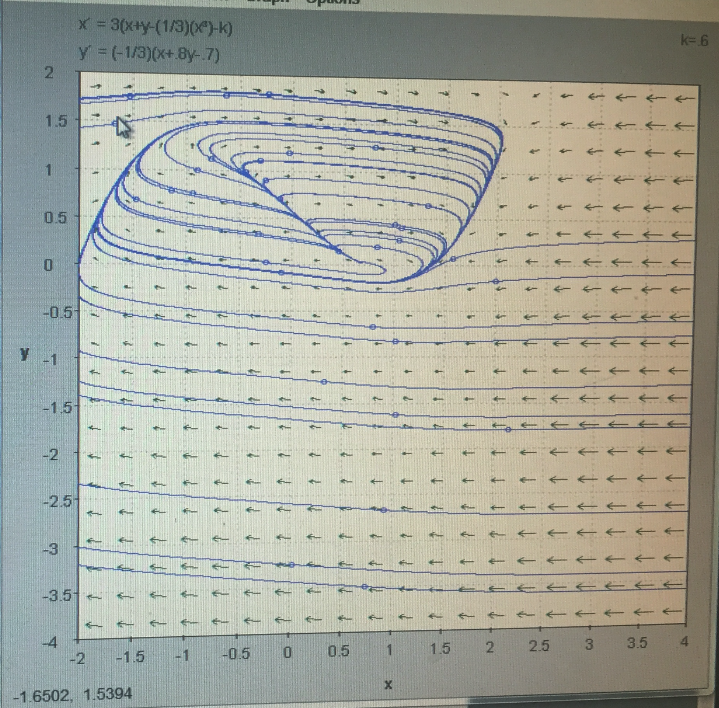# Ordinary Differential EquationsOrdinary differential equations (ODEs) are a branch of mathematics that deals with the study of how functions change over time. They are built upon the foundation of calculus, and are an evolution of the themes of single variable calculus. ODEs are used to model a wide range of real-world problems, from simple mechanical systems to complex biological and economic systems.
At the core of ODEs is the concept of a derivative. Derivatives are used to describe how a function changes over time. In single variable calculus, we learn how to calculate the derivative of a function, and how to use derivatives to find the slope of a curve at a given point. In ODEs, we take this a step further by using derivatives to understand how a function changes over a range of values.
One of the most common examples of ODEs is the motion of a simple harmonic oscillator. This is a system that oscillates back and forth at a constant frequency, such as a pendulum or a spring. The motion of the oscillator can be described by a second-order ODE, which is a differential equation that involves the second derivative of the function.
Another example of ODEs is the spread of a disease through a population. This can be modeled using a system of differential equations, which describe how the number of infected individuals changes over time. These equations take into account factors such as the rate of transmission, the rate of recovery, and the number of individuals in the population.
ODEs are also used in the field of engineering to model the behavior of mechanical systems. For example, the motion of a car on a road can be described using a system of ODEs, which take into account factors such as the car’s velocity, the road’s slope, and the forces acting on the car.
In finance, ODEs are used to model the behavior of financial markets. For example, the Black-Scholes equation is a partial differential equation that describes how the price of an option changes over time. This equation is widely used in the field of finance, and is essential for understanding the behavior of options and other derivatives.
The understanding of ODEs allows one’s thinking to expand and increases one’s ability to solve different kinds of problems. ODEs are a powerful tool that allows us to model and understand a wide range of real-world phenomena, from simple mechanical systems to complex biological and economic systems. They are used in a wide range of fields, including physics, engineering, biology, and finance.
In conclusion, ODEs are a branch of mathematics that deals with the study of how functions change over time. They are built upon the foundation of calculus, and are an evolution of the themes of single variable calculus. ODEs are used to model a wide range of real-world problems, from simple mechanical systems to complex biological and economic systems. The understanding of ODEs allows one’s thinking to expand and increases one’s ability to solve different kinds of problems. They are a powerful tool that allows us to model and understand a wide range of real-world phenomena, from simple mechanical systems to complex biological and economic systems.